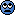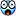# How is the colour of the avatar in PF is decided?

#### MathematicalPhysicist

Gold Member
the question in the title.

Related Feedback and Announcements News on Phys.org

#### Greg Bernhardt

Admin
As far as I know it's random. Obviously the background and letter colors are coordinated to keep contrast.

•jedishrfu

#### jedishrfu

Mentor
I found this on xenforo so it looks programmable

•Wrichik Basu

#### MathematicalPhysicist

Gold Member
As far as I know it's random. Obviously the background and letter colors are coordinated to keep contrast.
Nothing which is in a classical computer is truly random.
Though you can say that you don't know how is the colour chosen.

•DrClaude

#### DrClaude

Mentor
Nothing which is in a classical computer is truly random.
Though you can say that you don't know how is the colour chosen.
That's like saying that coin flips aren't really random because you could use Newton's laws to calculate on which side the coin will end up. Some results from computers can be random FAPP.

#### MathematicalPhysicist

Gold Member
That's like saying that coin flips aren't really random because you could use Newton's laws to calculate on which side the coin will end up. Some results from computers can be random FAPP.
And what's wrong with that?
If you know what the outcome will be how it can be random?
We don't actually know how to calculate this because of the unknown unknowns.

#### sysprog

And what's wrong with that?
If you know what the outcome will be how it can be random?
We don't actually know how to calculate this because of the unknown unknowns.
In @DrClaude's response, 'FAPP' abbreviates 'for all practical purposes'.

#### DrClaude

Mentor
If you know what the outcome will be how it can be random?
The point is that you can't know what the outcome will be. One could in theory dive in the code and try to figure out what the next color for an avatar will be, but even then that will probably depend on exactly how many new accounts have been created since the state of the program was checked. So FAPP no one knows, not even @Greg Bernhardt, what a particular new user's avatar's color will be. Therefore, it is random.

#### MathematicalPhysicist

Gold Member
In @DrClaude's response, 'FAPP' abbreviates 'for all practical purposes'.
I searched google for FAPP and got a colorful definition from the urban dictionary which won't be displayed here. So I was quite sure that wasn't what he meant... :-D

Last edited by a moderator:

#### sysprog

MathematicalPhysicist said:
So I was quite sure that wasn't what he meant... :-D
If you knew that @DrClaude didn't mean that, and you didn't confidently surmise what he did mean, you could have asked, and if you did know what he meant, there wasn't much good reason for you to take him to task over the distinction between web server random number generators that produce imperfectly random numbers that are nonetheless usably random, and numbers that are more completely random.

#### Greg Bernhardt

Admin
Nothing which is in a classical computer is truly random.
So you're looking for a quantum explanation for how the dynamic avatars are built?Let me take a look and see if I can find the code for it.

•Wrichik Basu

#### sysprog

So you're looking for a quantum explanation for how the dynamic avatars are built?Let me take a look and see if I can find the code for it.
I'm not a xenforo licensee, so I don't have access to the source code; however, if you're right about the color being 'random', presumably over a predefined pallette (the color and other characteristics can be overridden in css in file message.less, according to content of the discussion thread a link to which was posted by @jedishrfu), you would have to look at the server's php.ini file to ensure that php is getting its numbers from /dev/random, and then investigate the specifics of the implementation there, in order to get a reasonable idea of 'how random' the numbers generated really are, and the 'quantum explanation' would extend only to the ensourcement of the numbers in the /dev/random entropy pool.

•Wrichik Basu and jedishrfu

#### Greg Bernhardt

Admin
you would have to look at the server's php.ini file to ensure that php is getting its numbers from /dev/random, and then investigate the specifics of the implementation there, in order to get a reasonable idea of 'how random' the numbers generated really are, and the 'quantum explanation' would extend only to the ensourcement of the numbers in the /dev/random entropy pool.
Honestly I don't have time for all this. Best I can do is find the php code.•Wrichik Basu

#### sysprog

Honestly I don't have time for all this. Best I can do is find the php code.That's in itself far beyond any reasonable call of duty.#### Greg Bernhardt

Admin
Here is the php code (at least most of it)

PHP:
protected function getDefaultAvatarHtml($username,$innerClassHtml, array &$outerAttributes) {$styling = $this->getDefaultAvatarStyling($username);

if (empty($outerAttributes['style'])) {$outerAttributes['style'] = '';
}
else
{
$outerAttributes['style'] .= '; '; }$outerAttributes['style'] .= "background-color: $styling[bgColor]; color:$styling[color]";

if (empty($outerAttributes['class'])) {$outerAttributes['class'] = '';
}
else
{
$outerAttributes['class'] .= ' '; }$outerAttributes['class'] .= 'avatar--default avatar--default--dynamic';

return '<span class="' . $innerClassHtml . '">' .$styling['innerContent'] . '</span>';
}

protected function getDefaultAvatarStyling($username) { if (!isset($this->avatarDefaultStylingCache[$username])) {$bytes = md5($username, true);$r = dechex(round(5 * ord($bytes) / 255) * 0x33);$g = dechex(round(5 * ord($bytes) / 255) * 0x33);$b = dechex(round(5 * ord($bytes) / 255) * 0x33);$hexBgColor = sprintf('%02s%02s%02s', $r,$g, $b);$hslBgColor = \XF\Util\Color::hexToHsl($hexBgColor);$bgChanged = false;
if ($hslBgColor > 60) {$hslBgColor = 60;
$bgChanged = true; } else if ($hslBgColor < 15)
{
$hslBgColor = 15;$bgChanged = true;
}

if ($hslBgColor > 85) {$hslBgColor = 85;
$bgChanged = true; } else if ($hslBgColor < 15)
{
$hslBgColor = 15;$bgChanged = true;
}

if ($bgChanged) {$hexBgColor = \XF\Util\Color::hslToHex($hslBgColor); }$hslColor = \XF\Util\Color::darkenOrLightenHsl($hslBgColor, 35);$hexColor = \XF\Util\Color::hslToHex($hslColor);$bgColor = '#' . $hexBgColor;$color = '#' . $hexColor; if (preg_match($this->avatarLetterRegex, $username,$match))
{
$innerContent = htmlspecialchars(utf8_strtoupper($match));
}
else
{
$innerContent = '?'; }$this->avatarDefaultStylingCache[$username] = [ 'bgColor' =>$bgColor,
'color'        => $color, 'innerContent' =>$innerContent
];
}

return $this->avatarDefaultStylingCache[$username];
}

••mfb and sysprog

#### jedishrfu

Mentor
@MathematicalPhysicist lets not get hung up here on the notion of random.

Basically when we don't know how something is done it may appear random. However, further testing may reveal a pattern and eventually we learn something new. In the case of these default avatars it appears that they select the color scheme from a table so as to not place two similar colors for text and background.

I did a brief check via our "at"+username feature to see if I could see a pattern and I could not.

At first, I thought it might be:
- based on the first letter - no
- based on the first n letters - no

Leaving these two choices:
- based on a hash of the name
- based on random selection

These choices could be tested by starting with a default avatar noting its color scheme and then changing to a new avatar and then changing back. If you get the same avatar then its likely not random but based on some has of the name and/or or data in your description.

It is true that computer software cannot generate truly random without some hardware assist. However for all practical purposes the random number generators in computer software today are pretty good approximations for random numbers. In general, the random seed that initializes the generator code comes from the clock microseconds. You can however for code testing select a known seed and generate a repeatable list of "random" numbers.

https://en.wikipedia.org/wiki/Random_seed

These algorithms go through a lot of extensive testing as user generated algorithms tend to have a bias that comes out in the statistics taken of the sequence. Years ago, a math programmer at GE discovered for one such sequence that if you took the numbers generated as triplets and plotted them in 3D space they would all appear on a tilted plane indicating that given the first few numbers you could predict the next number. You would probably never notice a pattern like this but an implicit bias would occur in your results due to the generation of poorly constructed pseudo-random numbers.

You can learn more here at Khan Academy:

https://www.khanacademy.org/computing/computer-science/cryptography/crypt/v/random-vs-pseudorandom-number-generators

Lastly, UUIDs utilize a form of pseudo-random generation based on the system clock to ensure that all numbers generated across all computers are unique for all practical purposes:

https://en.wikipedia.org/wiki/Universally_unique_identifier

https://towardsdatascience.com/are-uuids-really-unique-57eb80fc2a87

I can't wait for that hapless programmer who has to debug a duplicate occurrence of UUID causing a problem in his/her code. Chances are though it will be chalked up to a hiccup somewhere and glossed over since its unlikely to be reproducible.

•sysprog

#### sysprog

So the color is genned from the MD5 hash on the username, which is as unpredictable as the (unique) usernames are, and then filtered through some integers to keep the colors inside a determined set of ranges. That's close enough to random for purposes of the original inquiry in this thread. Thanks, @Greg Bernhardt, for finding and posting the code.

•jedishrfu and Greg Bernhardt

#### jedishrfu

Mentor
Thanks @Greg Bernhardt the answer is in the code they do an md5 hash of the username and pick out indexes for the colors in some color table.
exerpted from Greg's PHP code post:
            $bytes = md5($username, true);
$r = dechex(round(5 * ord($bytes) / 255) * 0x33);
$g = dechex(round(5 * ord($bytes) / 255) * 0x33);
$b = dechex(round(5 * ord($bytes) / 255) * 0x33);
$hexBgColor = sprintf('%02s%02s%02s',$r, $g,$b);

Last edited:

#### Wrichik Basu

Gold Member
2018 Award
Please don't tell me after a while that Greg's servers use Quantum computation to choose an avatar.Gold Member
2018 Award

#### jedishrfu

Mentor
Oops! It was a pseudo-random mistake, I swear.

••DrClaude and Wrichik Basu

#### sysprog

Oops! It was a pseudo-random mistake, I swear.
That means it will eventually happen again.#### MathematicalPhysicist

Gold Member
So you're looking for a quantum explanation for how the dynamic avatars are built?Let me take a look and see if I can find the code for it.
Dunno, I once learnt electronic devices and also still learn solid state physics.

The transistors that make our computers (the classical computers not the quantum computers), are based on Quantum Physics (that makes me wonder how different can a quantum computer be from classical computer if they are built on the same transistors, that's for another thread), but their outcomes are still a sequence of 0-1 which are determined classically there are no qubits there.

I genuinely thought there was a way to determine the colour.
I am asking since the same colour here in my avatar also appeared in the math.stackexchange, so I guess that's sort of a coincidence.

#### jedishrfu

Mentor
Probably not a coincidence as they may be using the same software as PF with different outward color schemes...

Often coincidences harbor hidden connections that weren't obvious at first sight. There was a story of an autographed children's book lost in the US appearing years later in a book reseller in France found by the now grown child who owned it. At first, it seemed to be a remarkable coincidence until you discover that the French nanny was a common link.

#### Greg Bernhardt

Admin
I am asking since the same colour here in my avatar also appeared in the math.stackexchange, so I guess that's sort of a coincidence.
You are using a custom image as an avatar though.

### Want to reply to this thread?

"How is the colour of the avatar in PF is decided?"

### Physics Forums Values

We Value Quality
• Topics based on mainstream science
• Proper English grammar and spelling
We Value Civility
• Positive and compassionate attitudes
• Patience while debating
We Value Productivity
• Disciplined to remain on-topic
• Recognition of own weaknesses
• Solo and co-op problem solving